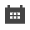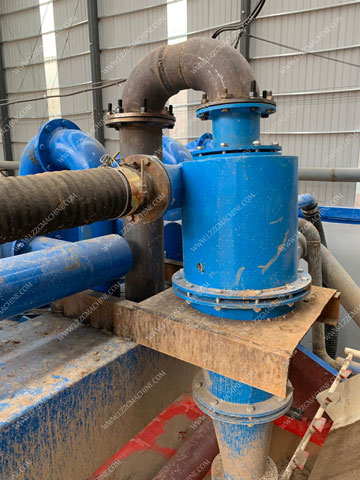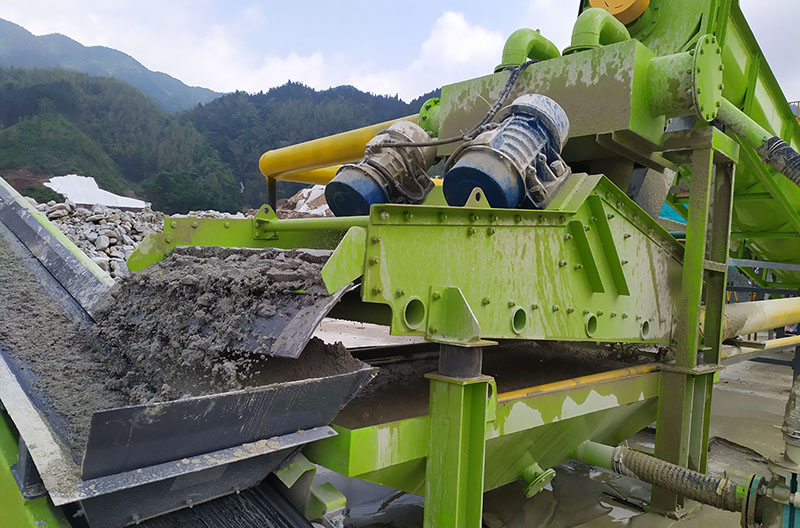# What particle size can a hydrocyclone separate?Hydrocyclone is a kind of grading equipment with a wide range of applications, widely used in non-ferrous metal beneficiation, chemical industry, ceramics and other industries. In addition to classifying materials, hydrocyclones can also perform desliming and concentration of materials.

## Classification particle size range of hydrocyclone

Compared with other classifiers, the grading particle size is finer, which is very suitable for the classification of fine-grained materials. When the grading particle size range is 0.3-0.01mm, the grading effect is better; the grading efficiency is higher, generally about 60%, sometimes it can be Up to 80~90%, 5-10% higher than the spiral classifier.### The model parameters of the hydrocyclone are as follows

FX-125, the overflow particle size is 20-50μm, the output is about 3.1-6.1m3 per hour, the overflow pipe diameter is 14, 18, 25, 35mm, the ore opening area is 25×10, and the sand discharge nozzle diameter is 15, 25mm ;

FX-150, the overflow particle size is 35-75μm, the hourly output is about 7.5-15m3, the overflow pipe diameter is 20, 40, 32, 25mm, the area of the ore inlet is 22×22, and the sand discharge nozzle diameter is 32, 24, 16mm;

FX-250, the overflow particle size is 40-100μm, the output is about 10-39m3 per hour, the overflow pipe diameter is 26, 34, 50, 69mm, the ore opening area is 50×20, and the sand discharge nozzle diameter is 20, 25, 35mm;

FX-300, the overflow particle size is 45-105μm, the output is about 37-43m3 per hour, the overflow pipe diameter is 65, 75mm, the ore opening area is 47×60, and the sand discharge nozzle diameter is 35, 40mm;

FX-350, the overflow particle size is 50-110μm, the output is about 74-90m3 per hour, the overflow pipe diameter is 115, 105, 95mm, the ore opening area is 80×65, and the sand discharge nozzle diameter is 80, 70, 60mm;

FX-500, the overflow particle size is 60-120μm, the output is about 170-220m3 per hour, the overflow pipe diameter is 180, 160, 140mm, the ore opening area is 110×120, and the sand discharge nozzle diameter is 110, 90, 70mm.Factors affecting hydrocyclone classification are

1. The diameter and height of the cylindrical cylinder. As this diameter increases, the handling capacity increases, but the overflow particle size becomes coarser, and vice versa. The height of the cylindrical cylinder affects the residence time of the pulp in the cyclone and the classification efficiency, but it is not good if it is too high or too low, generally 0.6-1.0 of the diameter.
2. Diameter of feed port. This diameter is usually 0.08-0.25 of the diameter of the cyclone. If it is larger than this value, the processing capacity can be increased, but the classification efficiency is low, and the ore opening is often made into a rectangle.
3. The diameter of the overflow pipe. Generally, it is 0.1-0.4 of the diameter of the cyclone, which can be used to adjust the relative productivity of overflow and sand settling. The diameter of the overflow pipe increases, the overflow volume increases, the overflow particle size becomes coarser, the fine particles in the settling sand decrease and the concentration increases.

How to choose the model of hydrocyclone?

When the user selects the type of hydrocyclone, there are two main bases: the volume of ore pulp to be processed Q (m3/h); the grading particle size d (mm) of the hydrocyclone determined by the previous experiment. Then determine the diameter D of the hydrocyclone based on these two points, and then use the diameter D to calculate and determine the diameter of the overflow pipe (d overflow), the diameter of the sand settling (d sinking), and the diameter of the ore feeding (d to ), cylinder height (H), overflow pipe insertion depth (h), cone angle (a): d overflow = (0.2 ~ 0.4) D, d sink = (0.2 ~ 0.7) d overflow, d give = (0.4 ～1.0)d overflow, H=(0.7～1.6)D, h=(0.5～0.8)H, a=10°-45°(The thicker the grading particle size is, the larger the cone angle a is, and vice versa). After these values are determined, the model size of the cyclone can basically be determined.

### Related Articles

Product Knowledge
Solutions
FAQ